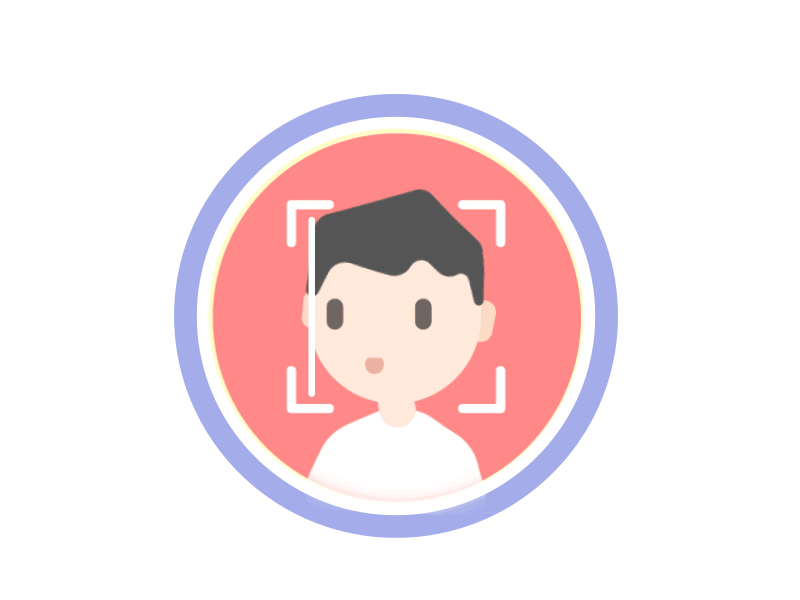Facial Recognition Using Open-CV

Source: Deep Learning on MediumFacial Recognition Using Open-CV

Face recognition is used for everything from automatically tagging pictures to unlocking cell phones. And with recent advancements in deep learning, the accuracy of face recognition has improved. In this project, we learnt how to develop a face recognition system that can detect faces and identify the faces.

The face recognition systems can operate basically in two modes:

◦Verification or authentication of a facial image: It compares the input facial image with the facial image related to the user which is requiring the authentication.

◦Facial Identification or facial recognition: It compares the input facial image with all facial images from a dataset to find the user that matches that face.

Coding Face Recognition using Python and OpenCV: We are going to divide the Face Recognition process in this project.

◦Prepare Training Data: Read training images for each person/subject along with their labels, detect faces from each image and assign each detected face an integer label of the person it belongs.

◦Train Face Recognizer: Train OpenCV’s LBPH recognizer by feeding it the data we prepared in step 1.

◦Prediction: Introduce some test images to face recognizer and see if it predicts them correctly.

ALGORITHM:

◦I used one of the old and more popular face recognition algorithms: Local Binary Patterns Histograms (LBPH).

◦As it is one of the easier face recognition algorithms and everyone can understand it without major difficulties.

◦It was first described in 1994 (LBP) and has since been found to be a powerful feature for texture classification.

Steps of the algorithm:

Parameters:

The LBPH uses 4 parameters:

Radius: The radius is used to build the circular local binary pattern and represents the radius around the central pixel. It is usually set to 1.

Neighbours: The number of sample points to build the circular local binary pattern. The higher the computational cost. It is usually set to 8.

Grid X: The number of cells in the horizontal direction. The more cells, the finer the grid, the higher the dimensionality of the resulting feature vector. It is usually set to 8.

Grid Y: The number of cells in the vertical direction. The more cells, the finer the grid, the higher the dimensionality of the resulting feature vector. It is usually set to 8.

Training the Algorithm:

First, we need to train the algorithm. To do so, we need to use a dataset with the facial images of the people we want to recognize. We need to also set an ID (it may be a number or the name of the person) for each image, so the algorithm will use this information to recognize an input image and give you an output. Images of the same person must have the same ID. With the training set already constructed.

Applying the LBP operation:

The first computational step of the LBPH is to create an intermediate image that describes the original image in a better way, by highlighting the facial characteristics. To do so, the algorithm uses a concept of a sliding window, based on the parameter’s radius and neighbours.

◦Suppose we have a facial image in grayscale.

◦We can get part of this image as a window of 3×3 pixels.

◦It can also be represented as a 3×3 matrix containing the intensity of each pixel (0~255).

◦Then, we need to take the central value of the matrix to be used as the threshold.

For each neighbour of the central value (threshold), we set a new binary value. We set 1 for values equal or higher than the threshold and 0 for values lower than the threshold.

◦Now, the matrix will contain only binary values (ignoring the central value). We need to concatenate each binary value from each position from the matrix line by line into a new binary value (e.g. 10001101).

◦Then, we convert this binary value to a decimal value and set it to the central value of the matrix, which is a pixel from the original image.

◦At the end of this procedure (LBP procedure), we have a new image which represents better the characteristics of the original image

The image below shows this procedure:

Extracting the Histograms: Now, using the image generated in the last step, we can use the Grid X and Grid Y parameters to divide the image into multiple grids, as can be seen in the following image:

◦Based on the image above, we can extract the histogram of each region as follows:

◦As we have an image in grayscale, each histogram (from each grid) will contain only 256 positions (0~255) representing the occurrences of each pixel intensity.

◦Then, we need to concatenate each histogram to create a new and bigger histogram. Supposing we have 8×8 grids, we will have 8x8x256=16.384 positions in the final histogram. The final histogram represents the characteristics of the image original image.

Performing face recognition:

In this step, the algorithm is already trained. Each histogram created is used to represent each image from the training dataset. So, given an input image, we perform the steps again for this new image and creates a histogram which represents the image.

◦So, to find the image that matches the input image we just need to compare two histograms and return the image with the closest histogram.

◦We can use various approaches to compare the histograms (calculate the distance between two histograms), for example, Euclidean distance, chi-square, absolute value, etc.

◦ In this example, we can use the Euclidean distance (which is quite known) based on the following formula:

Get the code on GitHub: https://github.com/PRATYUSHNAIR1976/Facial-Recognition-using-opencv